### Home > A2C > Chapter 5 > Lesson 5.1.2 > Problem5-29

5-29.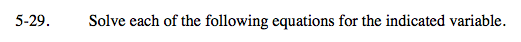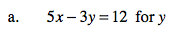Remember the strategies for solving algebraically.

To undo multiplication by 3, divide each term by 3.

$\textit{y}=\frac{3}{5}\textit{x}-4$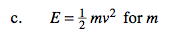$\text{To undo multiplication by } \frac{1}{2}$

(which is the same as dividing by 2), multiply both sides by 2.

To undo multiplication by v2, divide both sides by v2.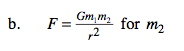To undo division by r2, multiply both sides by r2.

To undo multiplication by Gm1 divide both sides by Gm1.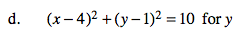To undo addition of (x − 4)2, subtract (x − 4)2 from both sides.

To undo squaring, take the square root of both sides. (Remember ±.)

To undo subtracting 1, add 1 to both sides. Remember to put it outside the radical sign.

$\textit{y}=\pm\sqrt{10-(\textit{x}-4)^{2}}+1$